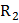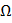# An unknown resistanceis connected in series with a resistance of 10. This combination is connected to one gap of meter bridge while a resistanceis connected in the other gap. The balance point is at 50 cm, Now, when the 10resistance is removed the balance point shifts 40cm. The value of(in ohm) is

## Question ID - 151361 :- An unknown resistanceis connected in series with a resistance of 10. This combination is connected to one gap of meter bridge while a resistanceis connected in the other gap. The balance point is at 50 cm, Now, when the 10resistance is removed the balance point shifts 40cm. The value of(in ohm) is

3537

The balance condition of a meter bridge experimentHere,Ist caseIInd caseSo, Eqs. (i) and (ii) giveNext Question :
 A wire has a resistance of 6. It is cut into two parts and both half values are connected in parallel. The new resistance is a) 3b) 6c) 12d) 1.5